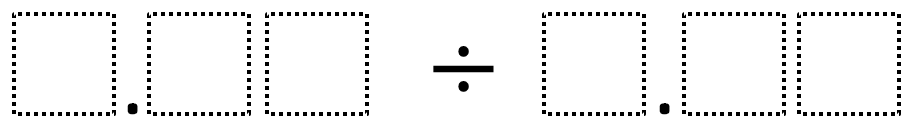Home > Grade 5 > Dividing Decimals (Elementary)

# Dividing Decimals (Elementary)

Directions: Using the digits 1 to 9 at most one time each, fill in the boxes to make the smallest (or largest) quotient.### Hint

What number does each box represent?

The current smallest quotient is 1.23 / 9.87 = 0.124. No answers yet for the largest quotient.  If you have one, post it in the comments.

Source: Robert Kaplinsky

## Multiplying Fractions 4

Directions: Using the digits 1 to 9 at most once each time, fill the boxes …

1.Just a heads up on a typo: quotient, not sum

•Good catch! Thanks. I’ll change it now.

2.1.23 / 9.87 = 0.124

•Thanks DYMB! I added it to the problem.

•Ashley McCullough

My students got this today, too! We had a great discussion about thinking of this problem as a fraction instead of a division problem. Once a student got 0.5 as an answer, other students realized they could get 1/4 by making the dividend a quarter of the divisor. That led to 1/8. So fun!!

3.9.87/1.23=8.024

4.My problem with either largest or smallest quotient is that your quotient repeats digits that were part of the divisor.

•Hi james it’s me thanks for helping me at school.

•Hi james it’s me thanks for helping me at school.

•Hi james it’s me thanks for helping me at school.WHY IS THIS A DUPLICATE HOW??!

•Hi james it’s me thanks for helping me at school.WHY IS THIS A DUPLICATE HOW??!

•Hi james it’s me thanks for helping me at school.WHY IS THIS A DUPLICATE HOW??!
Gfgfdbfdbfdfbdfdbfbd

5.largest is 9.87/1.23 = 8.0243902439

6.1.23 / 9.87 = 0.124

7.1.23 divided 9.87 = 0.124